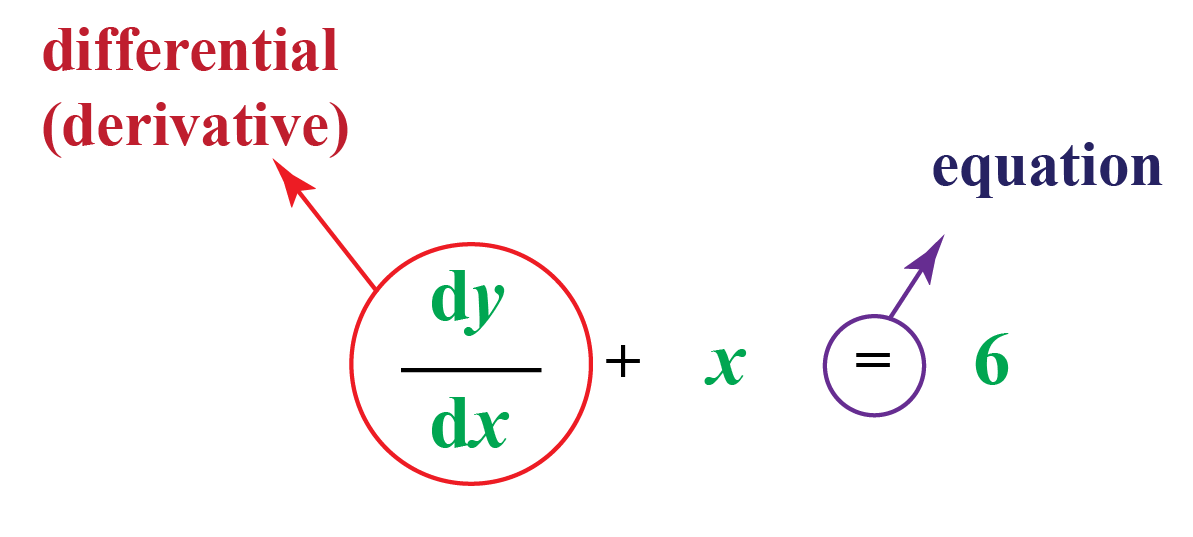# Differential Equation

Differential Equation
Go back to  'Calculus'

In this section, we will explore the world of differential equations. We will do so by learning about differential equation formulas, methods to solve them, and their applications. You can check out the interactive examples to know more about the lesson and try your hand at solving a few interesting practice questions at the end of the page.

A mathematical equation that includes variables such as x or y, as well as the rate at which those variables change, is a differential equation.Differential equations are unique since a function rather than a number is itself the solution of a differential equation.

## What Is Meant by Differential Equation?

An equation that contains the derivative of a function is called a differential function.

A differential equation is an equation that involves the derivative (derivatives) of the dependent variable with respect to the independent variable (variables) is called a differential equation.

The following are a few differential examples based on trignometric identities and other mathematical concepts.

$$\frac{{dy}}{{dx}} = \sin x$$

$$\frac{{d^2y}}{{dx^2}} + k^2y = 0$$

$$\frac{{d^2y}}{{dt^2}} + \frac{{d^2x}}{{dt^2}} = x$$

$$\frac{{d^3y}}{{dx^3}} + x\frac{{dy}}{{dx}} - 4xy = 0$$

$$\frac{{rdr}}{{d\theta }} + \cos \theta = 5$$

## What Are Different Types of Differential Equations?

### Homogenous Differential Equation

A differential equation in which the degree of all the terms is the same is known as a homogenous differential equation.

Example

1. $$y + x\frac{{dy}}{{dx}} = 0$$ is a homogenous differential equation of degree 1.

2. $$x^4 + y^4\frac{{dy}}{{dx}} = 0$$ is a homogenous differential equation of degree 4.

3. $$xy\frac{{dy}}{{dx}} + y^2 + 2x = 0$$ is not a homogenous differential equation.

### Linear Differential Equation

The differential equation of the form $$\frac{{dy}}{{dx}} + Py = Q$$ (Where P and Q are functions of x) is called linear differential equation.

$$\frac{{dy}}{{dx}} + Py = Q$$ (Where P, Q are constant or functions of y)

The general solution is $$y\times (I.F.)$$ = $$\int {Q(I.F.)dx + c}$$ where, I.F(integrating factor) = $$e^\int {^p{^d{^x}}}$$

### Partial Differential Equation

A differential equation that involves partial derivatives is known as a partial differential equation.

Example: $$\frac{{dy}}{{dx}} + x\frac{{dy}}{{dx}} = \frac{{y - t}}{{y + t}}$$

The above differential equation example is a partial differential equation since y  is a function of the two variables x and t, and partial derivatives are present.

### Ordinary Differential Equation

A differential equation that does not involves partial derivatives is known as a partial differential equation.

Example: $$\frac{{d^2y}}{{dx^2}} + \frac{{dy}}{{dx}} = 3y\cos x$$

The above differential equation example is an ordinary differential equation since it does not contain partial derivatives.

## How to Find Solution of Differential Equations?

A solution which can be obtained from the general solution by giving particular values to arbitrary constants is called a particular solution.

Therefore the differential equation has infinitely many solutions.

Let us understand differential equation solver by one example

$$\frac{{dy}}{{dx}} = x^2y + y$$

Step 1: Divide above differential equation by y.

$$\frac{1}{y}\frac{{dy}}{{dx}} = x^2 + 1$$

We consider $$y$$ and $$x$$ both as variables and write this as

$$\frac{{dy}}{y} = (x^2 + 1)dx$$

Step 2: Now integrate L.H.S. with respect to $$y$$ and with respect to $$x$$.

$$\int {\frac{1}{y}dx = \int {(x^2 + 1)dx} }$$

Step 3: After integrating, we get:

$$\log y = \frac{{x^3}}{3} + x + c$$

So, this is how differential equation is solved.

## What Are Orders of Differential Equation?

The order of a differential equation is the highest order of the derivative appearing in the equation.

Consider the following differential equations,

$$\frac{{dy}}{{dx}} = e^x$$, $$\frac{{d^4y}}{{dx^4}} + y = 0$$, $$(\frac{{d^3y}}{{dx^3}}) + x^2(\frac{{d^2y}}{{dx^2}}) = 0$$

In above differential equation examples, the highest derivative are of first, fourth and third order respectively.

## What Are Degrees of Differential Equation?

The degree of the differential equation is the power of the highest ordered derivative present in the equation.

To find the degree of the differential equation, we need to have a positive integer as the index of each derivative.

Consider the following differential equations examples.

$$(\frac{{d^3y}}{{dx^3}})^3 + 2(\frac{{d^2y}}{{dx^2}})^2 - \frac{{dy}}{{dx}} + y = 0$$

In the above differential equation, the highest order term is $$(\frac{{d^3y}}{{dx^3}})$$ and its power is 3, therefore the degree is 3.

$$(\frac{{dy}}{{dx}})^2 + \frac{{dy}}{{dx}} - \sin^2y = 0$$

In the above differential equation the highest order term is $$(\frac{{dy}}{{dx}})$$ and its power is 2, therefore the degree is 2.

More Important Topics
Numbers
Algebra
Geometry
Measurement
Money
Data
Trigonometry
Calculus